# vs.eyeandcontacts.com

## Chapter 13 Direct and Inverse Proportions Exercise 13.1

Question 1: Following are the car parking charges near a railway station upto
4 hours                       Rs 60
8 hours                       Rs 100
12 hours                     Rs 140
24 hours                     Rs 180
Check if the parking charges are in direct proportion to the parking time.
No, the parking charges are not in direct proportion to the parking time.

Question 2: A mixture of paint is prepared by mixing 1 part of red pigments with 8 parts of base. In the following table, find the parts of base that need to be added.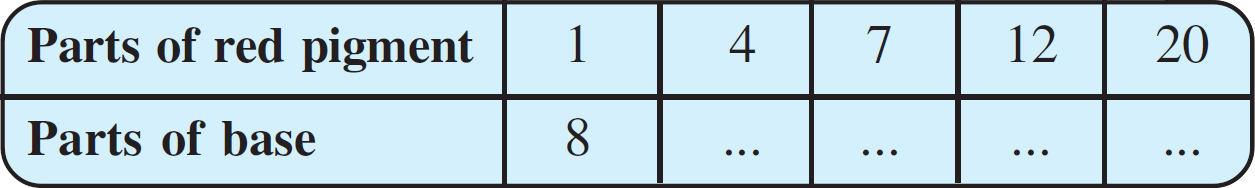Question 3: In Question 2 above, if 1 part of a red pigment requires 75 mL of base, how much red pigment should we mix with 1800 mL of base?
Let the red pigment that is required to be mixed with 1800 mL be x.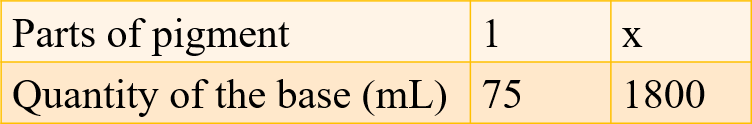= x₁/y₁ = x₂/y₂
= x₁y₂ = y₁x₂
= 1/75 = x/1800
= 75 × x = 1800 × 1
= x = 1800 × 1/75
= x = 24
Therefore, 24 parts of red pigment requires 1800 mL of base.

Question 4: A machine in a soft drink factory fills 840 bottles in six hours. How many bottles will it fill in five hours?
Let the number of bottles filled in hours be x.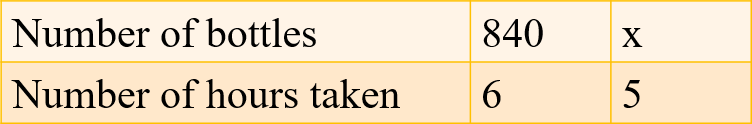= x₁/y₁ = x₂/y₂
= x₁y₂ = y₁x₂
= 840/6 = x/5
= 6 × x = 840 × 5
= x = 840 × 5/6
= x = 700
Therefore, 700 bottles will the machine fill in 5 hours.

Question 5: A photograph of a bacteria enlarged 50,000 times attains a length of 5 cm as shown in the diagram. What is the actual length of the bacteria? If the photograph is enlarged 20,000 times only, what would be its enlarged length?
Let the enlarged length of bacteria if the photograph is 20000 times only, be x.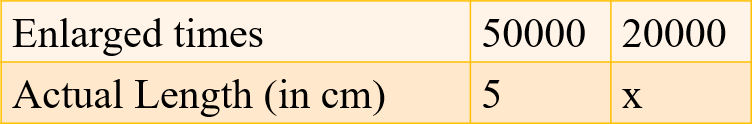= x₁/y₁ = x₂/y₂
= x₁y₂ = y₁x₂
= 50000/5 = 20000/x
= 50000 × x = 20000 × 5
= x = 20000 × 5/50000
= x = 2
Therefore, if the photograph is enlarged 20000 times only, 2 cm would be its enlarged length.

Question 6: In a model of a ship, the mast is 9 cm high, while the mast of the actual ship is 12 m high. If the length of the ship is 28 m, how long is the model ship?
Let the length of model ship be x.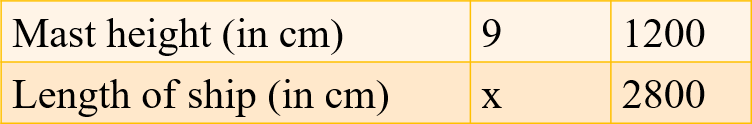= x₁/y₁ = x₂/y₂
= x₁y₂ = y₁x₂
= 9/x = 1200/2800
= 9 × 2800 = x × 1200
= x = 9 × 2800/1200
= x = 21
Therefore, the length of the model ship is 21 cm.

Question 7: Suppose 2 kg of sugar contains 9 × 106 crystals. How many sugar crystals are there in (i) 5 kg of sugar? (ii) 1.2 kg of sugar?
Let the number of sugar crystals in 5 kg sugar be x₁ and the number of sugar crystals in 1.2 kg of sugar be x₂.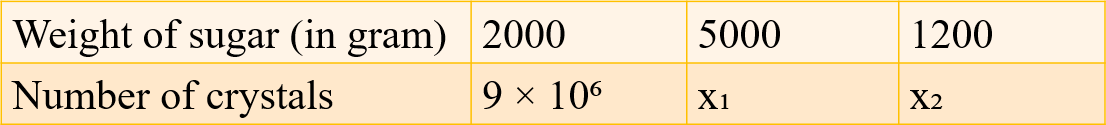i)
= x₁/y₁ = x₂/y₂
= x₁y₂ = y₁x₂
= 2000/9 × 10⁶ = 5000/x₁
= 2000 × x₁ = 9 × 10⁶ × 5000
= x₁ = 9 × 10⁶ × 5000/2000
= x₁ = 45000 × 10⁶/2000
= x₁ = 22.5 × 10⁶
= x₁ = 2.25 × 10⁷
Therefore, the number of sugar crystals in 5 kg sugar is 2.25 × 10⁷.

ii)
= x₁/y₁ = x₂/y₂
= x₁y₂ = y₁x₂
= 2000/9 × 10⁶ = 1200/x₂
= 2000 × x₂ = 9 × 10⁶ × 1200
= x₂ = 9 × 10⁶ × 1200/2000
= x₂ = 108 × 10⁶/20
= 54 × 10⁶/10
= 54 × 10⁵
= 5.4 × 10⁶
Therefore, the number of sugar crystals in 1.2 kg sugar is 5.4 × 10⁶.

Question 8: Rashmi has a road map with a scale of 1 cm representing 18 km. She drives on a road for 72 km. What would be her distance covered in the map?
Let the distance 72 km covered on the map be x.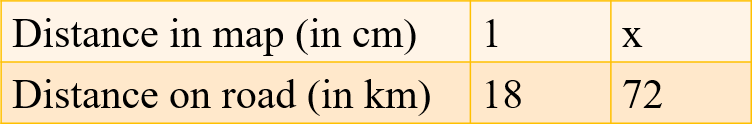= x₁/y₁ = x₂/y₂
= x₁y₂ = y₁x₂
= 1/18 = x/72
= 1 × 72 = x × 18
= x = 72/18
= x = 4
Therefore, the distance covered by Rashmi on the map would be 4 cm.

Question 9: A 5 m 60 cm high vertical pole casts a shadow 3 m 20 cm long. Find at the same time (i) the length of the shadow cast by another pole 10 m 50 cm high (ii) the height of a pole which casts a shadow 5m long.
Let the length of shadow cast by another pole 10 m 50 cm high be x₁. Let the height of a pole which casts a shadow 5 m long be x₂.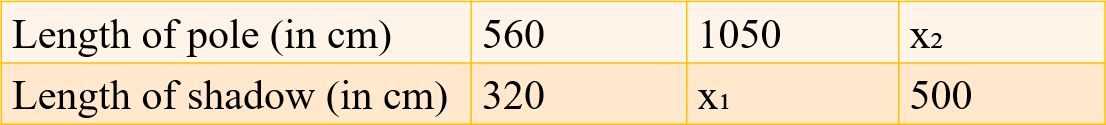i)
= x₁/y₁ = x₂/y₂
= x₁y₂ = y₁x₂
= 560/320 = 1050/x₁
= 560 × x₁ = 320 × 1050
= x₁ = 320 × 1050/560
= x₁ = 600
Therefore, the length of the shadow cast by another pole 10 m 50 cm high is 6 m (600 cm).

ii)
= x₁/y₁ = x₂/y₂
= x₁y₂ = y₁x₂
= 560/320 = x₂/500
= 560 × 500 = 320 × x₂
= x₂ = 560 × 500/320
= x₂ = 875
Therefore, the height of a pole which casts a shadow 5 m long is 8.75 m (875 cm).

Question 10: A loaded truck travels 14 km in 25 minutes. If the speed remains the same, how far can it travel in 5 hours?
Let the distance travelled by loaded truck in 5 hours be x.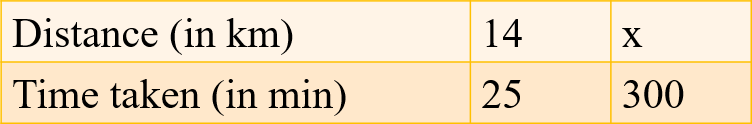= x₁/y₁ = x₂/y₂
= x₁y₂ = y₁x₂
= 14/25 = x/300
= 14 × 300 = 25 × x
= x = 14 × 300/25
= x = 168
Therefore, the loaded truck can travel 168 km in 5 hours.